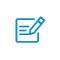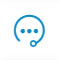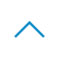# 模型剪枝，不可忽视的推断效率提升方法

本文来自可思数据(sykv.com)，转载请联系本站及注明出处

AlphaGo 使用了 1920 块 CPU 和 280 块 GPU，每场棋局光电费就需要 3000 美元。

可思数据-AI,sykv.com智能驾驶,人脸识别,区块链,大数据

本文来自可思数据(sykv.com)，转载请联系本站及注明出处

Christopher A Walsh. Peter Huttenlocher (1931–2013). Nature, 502(7470):172–172, 2013.

[Lecun et al. NIPS 89] [Han et al. NIPS 15]

https://github.com/tensorflow/model-optimization/blob/master/tensorflow_model_optimization/g3doc/guide/pruning/pruning_with_keras.ipynb

f = h5py.File("model_weights.h5",'r+')
for k in [.25, .50, .60, .70, .80, .90, .95, .97, .99]:
ranks = {}
for l in list(f[『model_weights』])[:-1]:
data = f[『model_weights』][l][l][『kernel:0』]
w = np.array(data)
ranks[l]=(rankdata(np.abs(w),method= 'dense')—1).astype(int).reshape(w.shape)
lower_bound_rank = np.ceil(np.max(ranks[l])*k).astype(int)
ranks[l][ranks[l]<=lower_bound_rank] = 0
ranks[l][ranks[l]>lower_bound_rank] = 1
w = w*ranks[l]
data[…] = w

f = h5py.File("model_weights.h5",'r+')
for k in [.25, .50, .60, .70, .80, .90, .95, .97, .99]:
ranks = {}
for l in list(f['model_weights'])[:-1]:
data = f['model_weights'][l][l]['kernel:0']
w = np.array(data)
norm = LA.norm(w,axis=0)
norm = np.tile(norm,(w.shape,1))
ranks[l] = (rankdata(norm,method='dense')—1).astype(int).reshape(norm.shape)
lower_bound_rank = np.ceil(np.max(ranks[l])*k).astype(int)
ranks[l][ranks[l]<=lower_bound_rank] = 0
ranks[l][ranks[l]>lower_bound_rank] = 1
w = w*ranks[l]
data[…] = w

可思数据-数据挖掘,智慧医疗,机器视觉,机器人sykv.com

本文来自可思数据(sykv.com)，转载请联系本站及注明出处

To prune, or not to prune: exploring the efficacy of pruning for model compression, Michael H. Zhu, Suyog Gupta, 2017（https://arxiv.org/pdf/1710.01878.pdf）
Learning to Prune Filters in Convolutional Neural Networks, Qiangui Huang et. al, 2018（https://arxiv.org/pdf/1801.07365.pdf）
Pruning deep neural networks to make them fast and small（https://jacobgil.github.io/deeplearning/pruning-deep-learning）扫码入群咨询反馈返回顶部